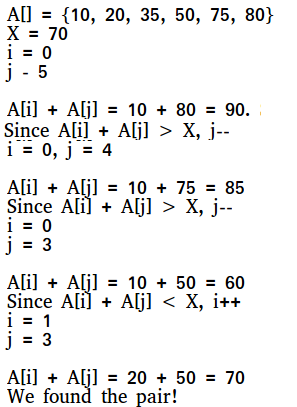Open In App

# C Program for Two Pointers Technique

Two pointers is really an easy and effective technique which is typically used for searching pairs in a sorted array.
Given a sorted array A (sorted in ascending order), having N integers, find if there exists any pair of elements (A[i], A[j]) such that their sum is equal to X.

Let’s see the naive solution.

## C

 `// Naive solution to find if there is a``// pair in A[0..N-1] with given sum.``#include `` ` `int` `isPairSum(``int` `A[],``int`  `N,``int` `X)``{``    ``for` `(``int` `i = 0; i < N; i++) {``        ``for` `(``int` `j = 0; j < N; j++) ``        ``{``            ``// as equal i and j means same element``            ``if` `(i == j) ``                ``continue``;``           ` `            ``// pair exists``            ``if` `(A[i] + A[j] == X)``                ``return` `true``; `` ` `            ``// as the array is sorted``            ``if` `(A[i] + A[j] > X)``                ``break``; ``        ``}``    ``}`` ` `    ``// No pair found with given sum.``    ``return` `0;``}`` ` `// Driver Code``int` `main()``{``    ``int` `arr[]={3,5,9,2,8,10,11};``    ``int` `val=17;``    ``int` `arrSize = ``sizeof``(arr)/``sizeof``(arr);``   ` `    ``// Function call``    ``printf``(``"%d"``,isPairSum(arr,arrSize,val));`` ` `    ``return` `0;``}`

Output

`1`

Time Complexity:  O(n2).

Now let’s see how the two-pointer technique works. We take two pointers, one representing the first element and other representing the last element of the array, and then we add the values kept at both the pointers. If their sum is smaller than X then we shift the left pointer to right or if their sum is greater than X then we shift the right pointer to left, in order to get closer to the sum. We keep moving the pointers until we get the sum as X.

## C

 `#include `` ` `// Two pointer technique based solution to find``// if there is a pair in A[0..N-1] with a given sum.``int` `isPairSum(``int` `A[], ``int` `N, ``int` `X)``{``    ``// represents first pointer``    ``int` `i = 0;`` ` `    ``// represents second pointer``    ``int` `j = N - 1;`` ` `    ``while` `(i < j)``    ``{``        ``// If we find a pair``        ``if` `(A[i] + A[j] == X)``            ``return` `1;`` ` `        ``// If sum of elements at current``        ``// pointers is less, we move towards``        ``// higher values by doing i++``        ``else` `if` `(A[i] + A[j] < X)``            ``i++;`` ` `        ``// If sum of elements at current``        ``// pointers is more, we move towards``        ``// lower values by doing j--``        ``else``            ``j--;``    ``}``    ``return` `0;``}`` ` `// Driver code``int` `main()``{``    ``// array declaration``    ``int` `arr[] = { 3, 5, 9, 2, 8, 10, 11 };``     ` `    ``// value to search``    ``int` `val = 17;``     ` `    ``// size of the array``    ``int` `arrSize = ``sizeof``(arr) / ``sizeof``(arr);``   ` `    ``// Function call``    ``printf``(``"%d"``, isPairSum(arr, arrSize, val));`` ` `    ``return` `0;``}`

Output

`1`

Illustration :Time Complexity:  O(n)

How does this work?
The algorithm basically uses the fact that the input array is sorted. We start the sum of extreme values (smallest and largest) and conditionally move both pointers. We move left pointer i when the sum of A[i] and A[j] is less than X. We do not miss any pair because the sum is already smaller than X. Same logic applies for right pointer j.

More problems based on two pointer technique.

Please refer complete article on Two Pointers Technique for more details!